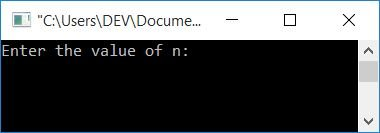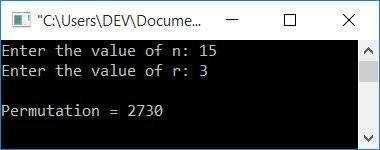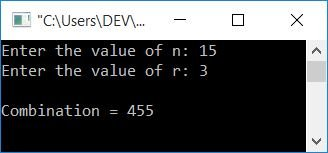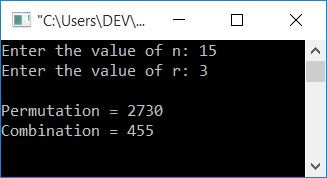# C Program to Find nCr and nPr

In this article, you will learn and get code for finding nCr (Combination) and nPr (Permutation) using a C program. But before going through the program, let's first understand its formula.

### nPr Formula

Let's suppose there are n players, for which we have to arrange r players. Therefore, to arrange r things out of n, use the permutation formula:

`nPr = n!/(n-r)!`

Note: The permutation value shows the ways to arrange r things out of n.

### nCr Formula

Let's suppose there are n number of players, from which we have to select r number of players. Therefore, to select r things out of n, use the combination formula:

`nCr = n!/r!(n-r)!`

Note: The combination value shows the ways to select r things out of n.

## Find nCr and nPr in C

In C programming, you must ask the user to enter the values of n and r to find the nCr and nPr values, as shown in the program below. Let's first start with finding nPr in C, then create another program that finds and prints the nCr value.

### C program to find nPr

The following programs receive the values of n and r from the user at program runtime and find and print the permutation value (nPr) on the output console:

```#include<stdio.h>
#include<conio.h>
int main()
{
long long fact=1, numerator, denominator;
int perm, n, r, i=1, sub;
printf("Enter the value of n: ");
scanf("%d", &n);
printf("Enter the value of r: ");
scanf("%d", &r);
while(i<=n)
{
fact = i*fact;
i++;
}
numerator=fact;                    // n!
sub = n-r;
fact=1;
i=1;
while(i<=sub)
{
fact=i*fact;
i++;
}
denominator = fact;                // (n-r)!
perm = numerator/denominator;
printf("\nPermutation = %d", perm);
getch();
return 0;
}```

This program was built and runs under the Code::Blocks IDE. Here is its sample run:Now supply the value of n, say 15, and then the value of r, say 3. To find the total number of ways to arrange 3 things out of 15 as shown below:The statement:

```while(i<=n)
{
fact = i*fact;
i++;
}```

is used to find the factorial of n. Later, a similar statement is used to find the factorial of sub (that equals n-r). The factorial of n is initialized to the numerator, and the factorial of sub or (n-r) is initialized to the denominator variables, respectively. Using the permutation formula as given above, just divide it and initialize the value to perm. Finally, print perm's value as a permutation value on output.

### C program to find nCr

Here is another program to find the combination (nCr):

```#include<stdio.h>
#include<conio.h>
int main()
{
long long fact=1, numerator, denominator;
int comb, n, r, i=1, sub;
printf("Enter the value of n: ");
scanf("%d", &n);
printf("Enter the value of r: ");
scanf("%d", &r);
while(i<=n)
{
fact = i*fact;
i++;
}
numerator=fact;                    // n!
sub = n-r;
fact=1;
i=1;
while(i<=sub)
{
fact=i*fact;
i++;
}
denominator = fact;                // (n-r)!
fact = 1;
i=1;
while(i<=r)
{
fact = i*fact;
i++;
}
denominator = (fact*denominator);
comb = numerator/denominator;
printf("\nCombination = %d", comb);
getch();
return 0;
}```

Here is its sample run:In addition to the permutation program given above, here we have also calculated the factorial of r. Because the denominator in the previous program held the factorial of (n-r), we have initialized r!(n-r)! to the denominator here.And finally, using the combination formula, divide the numerator value by the denominator, that is, n!/r!(n-r)!, and initialize it to the comb variable. Print "comb" as a combination value on the output.

### Find nCr and nPr using the function

This is the last program on permutations and combinations. This program was created using a user-defined function to find the values of nCr and nPr.

```#include<stdio.h>
#include<conio.h>
long long findFact(int);
long long findPerm(int, int);
long long findComb(int, int);
int main()
{
int comb, perm, n, r;
printf("Enter the value of n: ");
scanf("%d", &n);
printf("Enter the value of r: ");
scanf("%d", &r);
perm = findPerm(n, r);
comb = findComb(n, r);
printf("\nPermutation = %d", perm);
printf("\nCombination = %d", comb);
getch();
return 0;
}
long long findFact(int num)
{
int i=1;
long long fact=1;
while(i<=num)
{
fact = i*fact;
i++;
}
return fact;
}
long long findPerm(int n, int r)
{
int sub;
long long numt, dent;
sub = n-r;
numt = findFact(n);
dent = findFact(sub);
return numt/dent;
}
long long findComb(int n, int r)
{
int sub;
long long numt, dent, temp;
sub = n-r;
numt = findFact(n);
temp = findFact(sub);
dent = temp*findFact(r);
return numt/dent;
}```

Here is its sample run:#### The same program in different languages

C Quiz

« Previous Program Next Program »